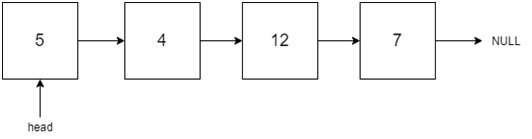# Partition List

Posted: 14 Mar, 2021
Difficulty: Easy

## PROBLEM STATEMENT

#### You are given a Singly Linked List of integers with a head pointer. Every node of the Linked List has a value written on it.#### Input Format:

``````The first line of the input contains an integer ‘T’ representing the number of test cases.

The first line of each test case contains space-separated integers denoting the values of nodes of the Linked List. The Linked List is terminated with -1. Hence, -1 is never a node value of the Linked List.

The second line of each test case contains a single integer ‘K’.
``````

#### Output Format:

``````For each test case return space-separated integers denoting the values of nodes of the Linked List.

The output of each test case will be printed in a separate line.
``````

#### Note:

``````You do not need to print anything, it has already been taken care of. Just implement the given function.
``````

#### Constraints:

``````1 <= T <= 5
0 <= N <= 5*10^4
1 <= DATA <= 10^9 and DATA != -1
1 <= K <= 10^9

Time Limit: 1 sec
``````Approach 1

The idea here is to Make two-pointers and store all nodes that have values less than ‘K’ in one pointer and all nodes that have values greater or equal to ‘K’ in another pointer. Then we will merge these two to get our answer.

Algorithm:

• Create two nodes that say ‘LESS’ and ‘GREATER’ to keep track of nodes that are less than ‘K’ and nodes that are greater than or equal to ‘K’
• Create two nodes, say ‘N1’ and ‘N2’ and initialize ‘N1’ with ‘LESS’ and ‘N2’ with ‘GREATER’.